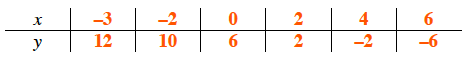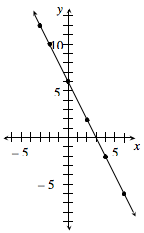### Home > MC2 > Chapter 6 > Lesson 6.1.5 > Problem6-48

6-48.

Use the graph below to add points to the table below.

For each point, find its $x$-coordinate and its $y$-coordinate and record the two numbers next to each other in the table.1. Write the rule in words.

Notice that for every $1$ the $x$-value increases, the $y$-coordinate decreases by $2$. Also take into account where the $y$-intercept is.

Multiply $x$ by $−2$ and then add $6$.

2. Explain how to use the table to predict the value of $y$ when $x$ is $–8$.

Extend the pattern in the table. Answers may vary.